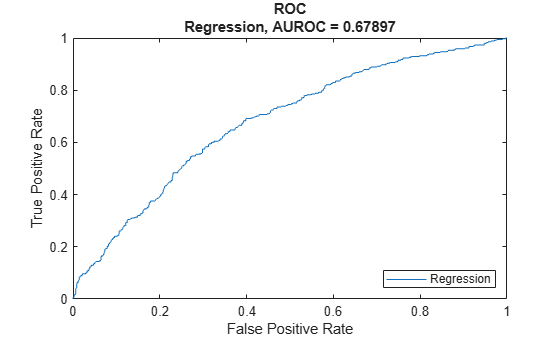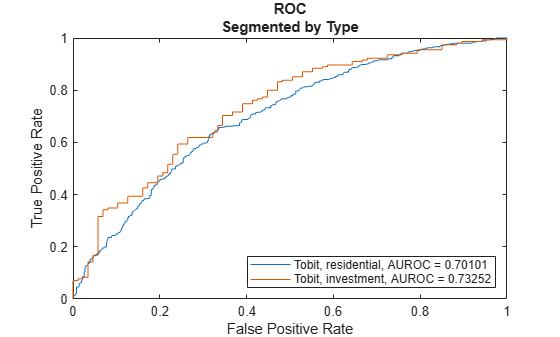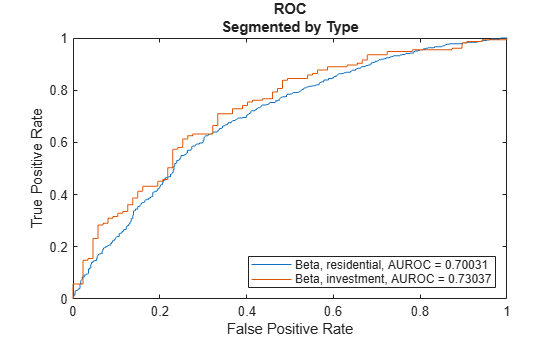# modelDiscrimination

Compute AUROC and ROC data

Since R2021a

## Syntax

``DiscMeasure = modelDiscrimination(lgdModel,data)``
``[DiscMeasure,DiscData] = modelDiscrimination(___,Name,Value)``

## Description

example

````DiscMeasure = modelDiscrimination(lgdModel,data)` computes the area under the receiver operating characteristic curve (AUROC). `modelDiscrimination` supports segmentation and comparison against a reference model and also alternative methods to discretize the LGD response into a binary variable.```

example

````[DiscMeasure,DiscData] = modelDiscrimination(___,Name,Value)` specifies options using one or more name-value pair arguments in addition to the input arguments in the previous syntax.```

## Examples

collapse all

This example shows how to use `fitLGDModel` to fit data with a `Regression` model and then use `modelDiscrimination` to compute AUROC and ROC.

Load the loss given default data.

```load LGDData.mat head(data)```
``` LTV Age Type LGD _______ _______ ___________ _________ 0.89101 0.39716 residential 0.032659 0.70176 2.0939 residential 0.43564 0.72078 2.7948 residential 0.0064766 0.37013 1.237 residential 0.007947 0.36492 2.5818 residential 0 0.796 1.5957 residential 0.14572 0.60203 1.1599 residential 0.025688 0.92005 0.50253 investment 0.063182 ```

Partition Data

Separate the data into training and test partitions.

```rng('default'); % for reproducibility NumObs = height(data); c = cvpartition(NumObs,'HoldOut',0.4); TrainingInd = training(c); TestInd = test(c);```

Create a `Regression` LGD Model

Use `fitLGDModel` to create a `Regression` model using training data. You can also use `fitLGDModel` to create a `Tobit` model by changing the `lgdModel` input argument to `'Tobit'`.

```lgdModel = fitLGDModel(data(TrainingInd,:),'Regression'); disp(lgdModel) ```
``` Regression with properties: ResponseTransform: "logit" BoundaryTolerance: 1.0000e-05 ModelID: "Regression" Description: "" UnderlyingModel: [1x1 classreg.regr.CompactLinearModel] PredictorVars: ["LTV" "Age" "Type"] ResponseVar: "LGD" ```

Display the underlying model.

`disp(lgdModel.UnderlyingModel)`
```Compact linear regression model: LGD_logit ~ 1 + LTV + Age + Type Estimated Coefficients: Estimate SE tStat pValue ________ ________ _______ __________ (Intercept) -4.7549 0.36041 -13.193 3.0997e-38 LTV 2.8565 0.41777 6.8377 1.0531e-11 Age -1.5397 0.085716 -17.963 3.3172e-67 Type_investment 1.4358 0.2475 5.8012 7.587e-09 Number of observations: 2093, Error degrees of freedom: 2089 Root Mean Squared Error: 4.24 R-squared: 0.206, Adjusted R-Squared: 0.205 F-statistic vs. constant model: 181, p-value = 2.42e-104 ```

Compute AUROC and ROC Data

Use `modelDiscrimination` to compute the AUROC and ROC for the test data set.

`[DiscMeasure,DiscData] = modelDiscrimination(lgdModel,data(TestInd,:),'ShowDetails',true)`
```DiscMeasure=1×3 table AUROC Segment SegmentCount _______ __________ ____________ Regression 0.67897 "all_data" 1394 ```
```DiscData=1395×3 table X Y T __________ _________ _______ 0 0 0.87604 0 0.0029326 0.87604 0 0.0058651 0.7515 0.00094967 0.0058651 0.44074 0.0018993 0.0058651 0.43569 0.0018993 0.0087977 0.40058 0.002849 0.0087977 0.31703 0.002849 0.01173 0.30375 0.002849 0.014663 0.28789 0.002849 0.017595 0.27996 0.0037987 0.017595 0.27026 0.0047483 0.017595 0.26868 0.005698 0.017595 0.26854 0.005698 0.020528 0.26682 0.0066477 0.020528 0.26668 0.0066477 0.02346 0.24923 ⋮ ```

You can visualize the ROC data using `modelDiscriminationPlot`.

`modelDiscriminationPlot(lgdModel,data(TestInd,:))`This example shows how to use `fitLGDModel` to fit data with a `Tobit` model and then use `modelDiscrimination` to compute AUROC and ROC.

Load the loss given default data.

```load LGDData.mat head(data)```
``` LTV Age Type LGD _______ _______ ___________ _________ 0.89101 0.39716 residential 0.032659 0.70176 2.0939 residential 0.43564 0.72078 2.7948 residential 0.0064766 0.37013 1.237 residential 0.007947 0.36492 2.5818 residential 0 0.796 1.5957 residential 0.14572 0.60203 1.1599 residential 0.025688 0.92005 0.50253 investment 0.063182 ```

Partition Data

Separate the data into training and test partitions.

```rng('default'); % for reproducibility NumObs = height(data); c = cvpartition(NumObs,'HoldOut',0.4); TrainingInd = training(c); TestInd = test(c);```

Create a `Tobit` LGD Model

Use `fitLGDModel` to create a `Tobit` model using training data.

```lgdModel = fitLGDModel(data(TrainingInd,:),'tobit'); disp(lgdModel) ```
``` Tobit with properties: CensoringSide: "both" LeftLimit: 0 RightLimit: 1 ModelID: "Tobit" Description: "" UnderlyingModel: [1x1 risk.internal.credit.TobitModel] PredictorVars: ["LTV" "Age" "Type"] ResponseVar: "LGD" ```

Display the underlying model.

`disp(lgdModel.UnderlyingModel)`
```Tobit regression model: LGD = max(0,min(Y*,1)) Y* ~ 1 + LTV + Age + Type Estimated coefficients: Estimate SE tStat pValue _________ _________ _______ __________ (Intercept) 0.058257 0.027276 2.1358 0.032809 LTV 0.20126 0.031373 6.415 1.7363e-10 Age -0.095407 0.0072543 -13.152 0 Type_investment 0.10208 0.018054 5.6542 1.7802e-08 (Sigma) 0.29288 0.005704 51.346 0 Number of observations: 2093 Number of left-censored observations: 547 Number of uncensored observations: 1521 Number of right-censored observations: 25 Log-likelihood: -698.383 ```

Compute AUROC and ROC Data

Use `modelDiscrimination` to compute the AUROC and ROC for the test data set.

`DiscMeasure = modelDiscrimination(lgdModel,data(TestInd,:),'ShowDetails',true,'SegmentBy',"Type",'DiscretizeBy',"median")`
```DiscMeasure=2×3 table AUROC Segment SegmentCount _______ _____________ ____________ Tobit, Type=residential 0.70101 "residential" 1152 Tobit, Type=investment 0.73252 "investment" 242 ```

You can visualize the ROC using `modelDiscriminationPlot`.

`modelDiscriminationPlot(lgdModel,data(TestInd,:),'SegmentBy',"Type",'DiscretizeBy',"median")`This example shows how to use `fitLGDModel` to fit data with a `Beta` model and then use `modelDiscrimination` to compute AUROC and ROC.

Load the loss given default data.

```load LGDData.mat head(data)```
``` LTV Age Type LGD _______ _______ ___________ _________ 0.89101 0.39716 residential 0.032659 0.70176 2.0939 residential 0.43564 0.72078 2.7948 residential 0.0064766 0.37013 1.237 residential 0.007947 0.36492 2.5818 residential 0 0.796 1.5957 residential 0.14572 0.60203 1.1599 residential 0.025688 0.92005 0.50253 investment 0.063182 ```

Partition Data

Separate the data into training and test partitions.

```rng('default'); % for reproducibility NumObs = height(data); c = cvpartition(NumObs,'HoldOut',0.4); TrainingInd = training(c); TestInd = test(c);```

Create a `Beta` LGD Model

Use `fitLGDModel` to create a risk_ug#object_model_beta_lgd model using training data.

```lgdModel = fitLGDModel(data(TrainingInd,:),'Beta'); disp(lgdModel) ```
``` Beta with properties: BoundaryTolerance: 1.0000e-05 ModelID: "Beta" Description: "" UnderlyingModel: [1x1 risk.internal.credit.BetaModel] PredictorVars: ["LTV" "Age" "Type"] ResponseVar: "LGD" ```

Display the underlying model.

`disp(lgdModel.UnderlyingModel)`
```Beta regression model: logit(LGD) ~ 1_mu + LTV_mu + Age_mu + Type_mu log(LGD) ~ 1_phi + LTV_phi + Age_phi + Type_phi Estimated coefficients: Estimate SE tStat pValue ________ ________ _______ __________ (Intercept)_mu -1.3772 0.13201 -10.433 0 LTV_mu 0.60269 0.15087 3.9947 6.7019e-05 Age_mu -0.47464 0.040264 -11.788 0 Type_investment_mu 0.45372 0.085143 5.3289 1.094e-07 (Intercept)_phi -0.16336 0.12591 -1.2974 0.19463 LTV_phi 0.055887 0.14719 0.37969 0.70421 Age_phi 0.22887 0.040335 5.6743 1.5864e-08 Type_investment_phi -0.14102 0.078155 -1.8044 0.07131 Number of observations: 2093 Log-likelihood: -5291.04 ```

Compute AUROC and ROC Data

Use `modelDiscrimination` to compute the AUROC and ROC for the test data set.

`DiscMeasure = modelDiscrimination(lgdModel,data(TestInd,:),'ShowDetails',true,'SegmentBy',"Type",'DiscretizeBy',"median")`
```DiscMeasure=2×3 table AUROC Segment SegmentCount _______ _____________ ____________ Beta, Type=residential 0.70031 "residential" 1152 Beta, Type=investment 0.73037 "investment" 242 ```

You can visualize the ROC using `modelDiscriminationPlot`.

`modelDiscriminationPlot(lgdModel,data(TestInd,:),'SegmentBy',"Type",'DiscretizeBy',"median")`## Input Arguments

collapse all

Loss given default model, specified as a previously created `Regression`, `Tobit`, or `Beta` object using `fitLGDModel`.

Data Types: `object`

Data, specified as a `NumRows`-by-`NumCols` table with predictor and response values. The variable names and data types must be consistent with the underlying model.

Data Types: `table`

### Name-Value Arguments

Specify optional pairs of arguments as `Name1=Value1,...,NameN=ValueN`, where `Name` is the argument name and `Value` is the corresponding value. Name-value arguments must appear after other arguments, but the order of the pairs does not matter.

Before R2021a, use commas to separate each name and value, and enclose `Name` in quotes.

Example: ```[DiscMeasure,DiscData] = modelDiscrimination(lgdModel,data(TestInd,:),'DataID','Testing','DiscretizeBy','median')```

Data set identifier, specified as the comma-separated pair consisting of `'DataID'` and a character vector or string. The `DataID` is included in the output for reporting purposes.

Data Types: `char` | `string`

Discretization method for LGD `data`, specified as the comma-separated pair consisting of `'DiscretizeBy'` and a character vector or string.

• `'mean'` — Discretized response is `1` if observed LGD is greater than or equal to the mean LGD, `0` otherwise.

• `'median'` — Discretized response is `1` if observed LGD is greater than or equal to the median LGD, `0` otherwise.

• `'positive'` — Discretized response is `1` if observed LGD is positive, `0` otherwise (full recovery).

• `'total'` — Discretized response is `1` if observed LGD is greater than or equal to `1` (total loss), `0` otherwise.

Data Types: `char` | `string`

Name of a column in the `data` input, not necessarily a model variable, to be used to segment the data set, specified as the comma-separated pair consisting of `'SegmentBy'` and a character vector or string. One AUROC is reported for each segment, and the corresponding ROC data for each segment is returned in the optional output.

Data Types: `char` | `string`

Since R2022a

Indicates if the output includes columns showing segment value and segment count, specified as the comma-separated pair consisting of `'ShowDetails'` and a scalar logical.

Data Types: `logical`

LGD values predicted for `data` by the reference model, specified as the comma-separated pair consisting of `'ReferenceLGD'` and a `NumRows`-by-`1` numeric vector. The `modelDiscrimination` output information is reported for both the `lgdModel` object and the reference model.

Data Types: `double`

Identifier for the reference model, specified as the comma-separated pair consisting of `'ReferenceID'` and a character vector or string. `'ReferenceID'` is used in the `modelDiscrimination` output for reporting purposes.

Data Types: `char` | `string`

## Output Arguments

collapse all

AUROC information for each model and each segment, returned as a table. `DiscMeasure` has a single column named `'AUROC'` and the number of rows depends on the number of segments and whether you use a `ReferenceID` for a reference model . The row names of `DiscMeasure` report the model IDs, segment, and data ID. If the optional `ShowDetails` name-value argument is `true`, the `DiscMeasure` output displays `Segment` and `SegmentCount` columns.

Note

If you do not specify `SegmentBy` and use `ShowDetails` to request the segment details, the two columns are added and show the `Segment` column as `"all_data"` and the sample size (minus missing values) for the `SegmentCount` column.

ROC data for each model and each segment, returned as a table. There are three columns for the ROC data, with column names `'X'`, `'Y'`, and `'T'`, where the first two are the X and Y coordinates of the ROC curve, and T contains the corresponding thresholds. For more information, see Model Discrimination or `perfcurve`.

If you use `SegmentBy`, the function stacks the ROC data for all segments and `DiscData` has a column with the segmentation values to indicate where each segment starts and ends.

If reference model data is given, the `DiscData` outputs for the main and reference models are stacked, with an extra column `'ModelID'` indicating where each model starts and ends.

collapse all

### Model Discrimination

Model discrimination measures the risk ranking.

The `modelDiscrimination` function computes the area under the receiver operator characteristic (AUROC) curve, sometimes called simply the area under the curve (AUC). This metric is between 0 and 1 and higher values indicate better discrimination.

To compute the AUROC, you need a numeric prediction and a binary response. For loss given default (LGD) models, the predicted LGD is used directly as the prediction. However, the observed LGD must be discretized into a binary variable. By default, observed LGD values greater than or equal to the mean observed LGD are assigned a value of 1, and values below the mean are assigned a value of 0. This discretized response is interpreted as "high LGD" vs. "low LGD." Therefore, the `modelDiscrimination` function measures how well the predicted LGD separates the "high LGD" vs. the "low LGD" observations. You can change the discretization criterion with the `DiscretizeBy` name-value pair argument.

To plot the receiver operator characteristic (ROC) curve, use the `modelDiscriminationPlot` function. However, if the ROC curve data is needed, use the optional `DiscData` output argument from the `modelDiscrimination` function.

The ROC curve is a parametric curve that plots the proportion of

• High LGD cases with predicted LGD greater than or equal to a parameter t, or true positive rate (TPR)

• Low LGD cases with predicted LGD greater than or equal to the same parameter t, or false positive rate (FPR)

The parameter t sweeps through all the observed predicted LGD values for the given data. The `DiscData` optional output contains the TPR in the `'X'` column, the FPR in the `'Y'` column, and the corresponding parameters t in the `'T'` column. For more information about ROC curves, see ROC Curve and Performance Metrics.

 Baesens, Bart, Daniel Roesch, and Harald Scheule. Credit Risk Analytics: Measurement Techniques, Applications, and Examples in SAS. Wiley, 2016.

 Bellini, Tiziano. IFRS 9 and CECL Credit Risk Modelling and Validation: A Practical Guide with Examples Worked in R and SAS. San Diego, CA: Elsevier, 2019.